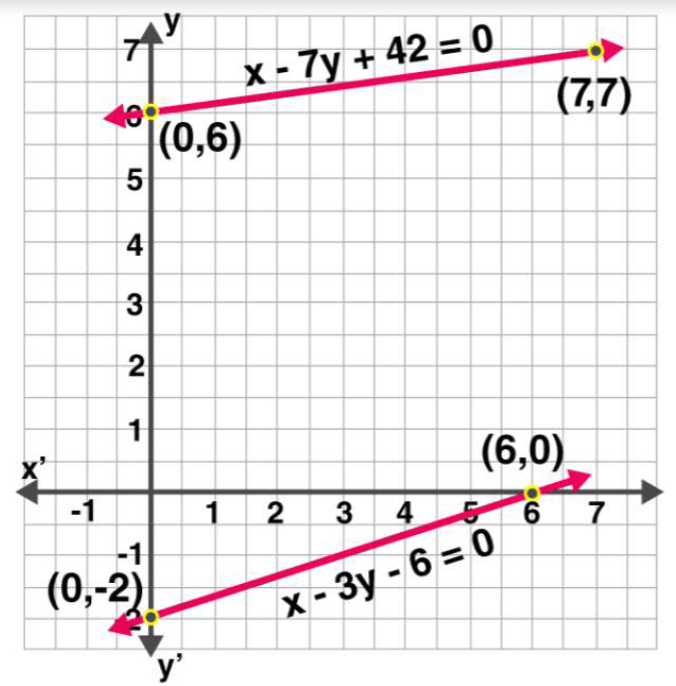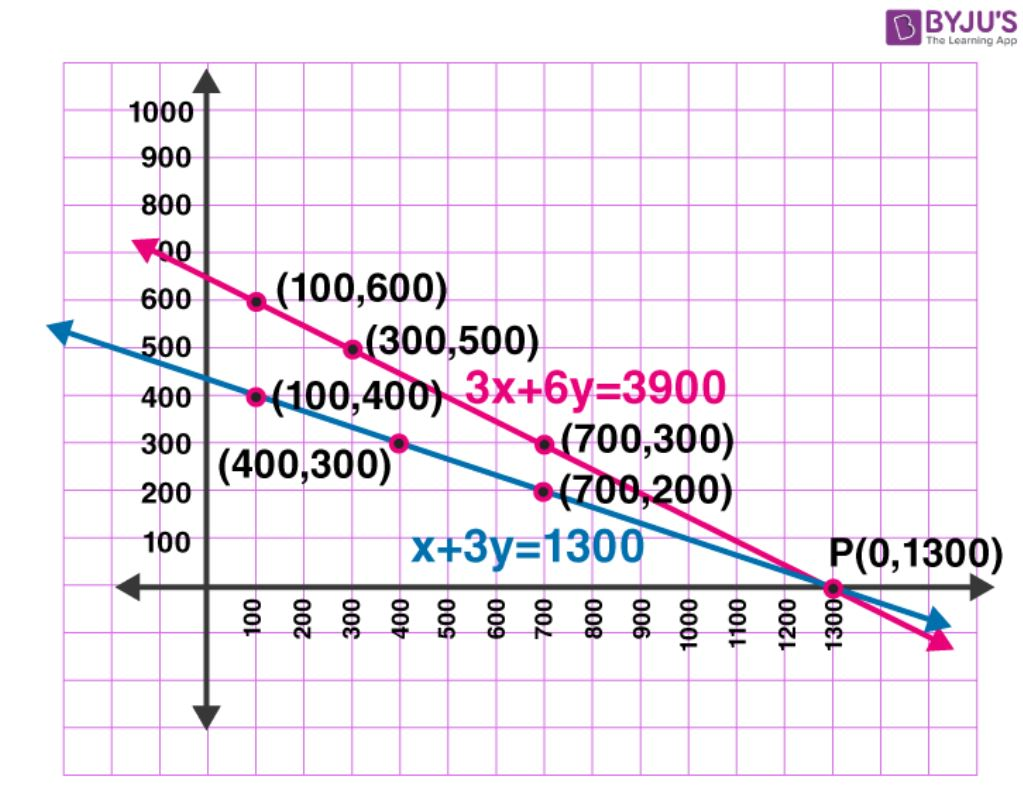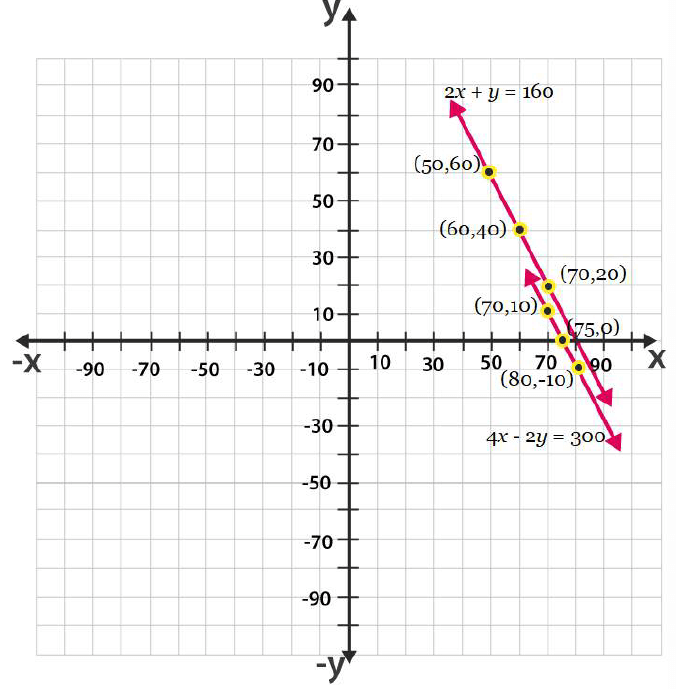### NCERT Solutions For Class 10 Math Chapter – 10 Exercise – 3.1

NCERT Solutions For Class 10 Math Chapter – 3 Exercise – 3.1Q1. Aftab tells his daughter “ Seven years ago, I was seven times as old as you were then Also , three years from now, I shall be three times as old you will be” Represent this situation algebraically and graphically.

Solution:-

A.T.Q

(x-7) = 7y – 49

X = 7y – 42

Putting y = 5 , 6 and 7 we get

X = 7 x 5 – 42 =

35 – 42 = -7

X = 7 x 6 – 42 = 42 – 42 = 0

X = 7 x 7 – 42 = 49 – 42  = 7

 x -7 0 7 y 5 6 7

A.T.Q

(x + 3) = 3(y + 3)

X = 3y + 6

Putting , y = -2 ,-1 and 0 , we get

X = 6

 x 0 3 6 y -2 -1 0

Algebraic representation

From equation (I) and (ii)

X – 7y = -42

X – 3y = 6

Graphical representation.Q2. The coach of a cricket team buys 3 bats and 6 balls for Rs 3900 Later she buys another bat and 3 more balls of the same kind for Rs 1300. Represent this situation algebraically and geometrically.

Solution:-

A.T.Q

3x + 6y = 3900 (i)

Dividing equation by 3 , we get

X + 2y = 1300

Subtracting 2y both side we get

X = 1300 – 2y

Putting y = -1300  , 0  and 1300 we get

x = 1300 – 2(-1300)

= 1300 + 2600

= 3900

X = 1300 – 2(0)

= 1300 – 0

= 1300

 x 3900 1300 -1300 y -1300 0 1300

X + 2y = 1300

X = 1300 – 2y

X = 1300 – 2(-1300)

= 1300 +  2600

= 3900

X = 1300 – 2(0)

= 1300 – 2(1300)

= -1300

 x 3900 1300 -1300 y -1300 0 1300

Algebraic representation

3x + 6y = 3900

X + 2y = 1300

Graphical representation,Q3. The cost of 2 kg of apples and 1 kg of grapes on a day was found to be Rs 160 After a month , the cost of 4 kg of apples and 2 kg of grapes is Rs 300. Represent the situation algebraically and geometrically.

Solution:-

2x + y = 160 (i)

2x = 160 – y

X = (160 – y)/2

X = 0

 x 80 40 0 y 0 80 160

4x + 2y =  300 (ii)

Dividing by 2 we get

2x + y = 150

Y = 150 – 2x

Y = 150 – 2 x 0

Y = 150

Y = 150 – 2 x 50 = 50

Y = 150 – 2 x (100) = – 50

 x 0 50 100 y 150 50 -50

Algebraic representation,

2x + y = 160 (i)

4x + 2y = 300 (ii)

Graphical representation,NCERT Solutions For Class 10 Math Chapter – 3 Exercise – 3.1     Q1. Aftab tells his daughter “ Seven…